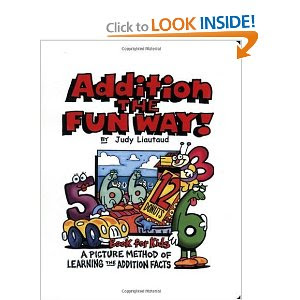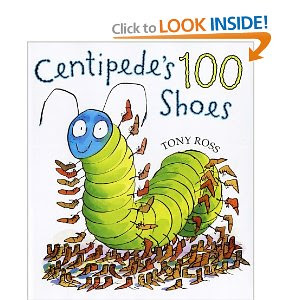## Tuesday, October 25, 2011

### Annotated Bib - Addition

Addition is a tool that people use frequently both as children and adults. Addition is the process of computing two or more whole numbers. Addition is commutative which means that the order of the problem does not change the sum or the outcome. Learning to add correctly at a young age is vital, and will be used almost daily.

Books

Addition The Fun Way! By Judy Liautaud. Illus. by Val Chadwick Bagley. 1996. 92p. City Creek Press, (97818833841348). Gr. K-2. This silly book allows children to learn math outside the box. "Addition the Fun Way" grabs the reader's attention as it uses interesting objects and characrters to explain basic addition concepts.Centipede's One Hundred Shoes . By Tony Ross. Illus. by the author. 2003. 32p. Henry Holt and Co., (9780805072983). Gr. K-2. This book is just as entertaining as it is educational. It offers a great deal to count as well as wonderfil illustrations that help hold a student's attention.Hershey's Kisses Addition Book . By Jerry Pallotta. Illus. by Rob Bolster. 2001. 32p. Scholastic, (9780439241731). Gr. K-2. Clowns and Hershey Kisses make addition fun! This book introduces basic addition concepts, and makes it interesting for young readers.

Ten Friends . By Bruce Goldstone. Illus. by Heather Cahoon. 2001.32p. Henry Holt and Co., (9780805062496). Gr. K-1. This book shows readers how many different ways can be used to count up to 10. The author uses different characters to help readers understand adding to 10 with different numerals.

The M&M Addition Book. By Barbara Barbieri McGrath. Illus. by the author. 2004. 32p.CharlesBridge Publishing, (9780881063493). Gr. K-2. This book takes a slightly different approach in teaching addition, but can still hold a readers attention. The candy characters helps make it an enjoyable read while simultaneously teaching addition as well.

Websites

Addition Speed Grid This site challenges you to answer a certain number of addition questions in a timed period. The website offers a grid, and allows you to chose from the grid to solve the problem.

Add It Up. This site gives you a variety of options to chose from. This allows a student to practice simple addition probelms with both numerals and objects.

Fun Brain Soccer Shootout. What can beat a fun competitive game of soccer addition?? This website offers a unique approach to addition. Answer the questions correctly and score a goal!

Math Magician. Reinforcment, practice, and mathematical magic gives this site an edge. It challenges participants to challenge themselves to add correctly while being timed.

More or Less. This site encourages students to practice and use one to one correspondence, subitizing, and conservation of numbers.

For Teachers

VA Standards of Learning
1.4 The student, given a familiar problem situation involving magnitude, will
a) select a reasonable order of magnitude from three given quantities: a one-digit numeral, a two-digit numeral, and a three-digit numeral (e.g., 5, 50, 500); and
b) explain the reasonableness of the choice.
1.5 The student will recall basic addition facts with sums to 18 or less and the corresponding subtraction facts.
1.6 The student will create and solve one-step story and picture problems using basic addition facts with sums to 18 or less and the corresponding subtraction facts.

Backround information from curriculum framework

• Associate the terms addition, adding, and sum with the concept of joining or combining.
• Provide practice in the use and selection of strategies. Encourage students to develop efficient strategies. Examples of strategies for developing the basic addition and subtraction facts include
– counting back;
– “one-more-than,” “two-more-than” facts;
– “one-less-than,” “two-less-than” facts;
– “doubles” to recall addition facts (e.g., 2 + 2 =__;
3 + 3 =__);
– “near doubles” [e.g., 3 + 4 = (3 + 3) + 1 = __];
– “make-ten” facts (e.g., at least one addend of 8 or 9);
– “think addition for subtraction” (e.g., for 9 – 5 = __, think “5 and what number makes 9?”);
– use of the commutative property, without naming the property (e.g., 4 +3 is the same as 3 + 4);
– use of related facts (e.g., 4 + 3 = 7 , 3 + 4 = 7, 7 – 4 = 3, and 7 – 3 = 4);
– use of the additive identity property (e.g., 4 + 0 = 4), without naming the property but saying, “When you add zero to a number, you always get the original number.”; and
– use patterns to make sums (e.g., 0 + 5 = 5,
1 + 4 = 5, 2 + 3 = 5, etc.).